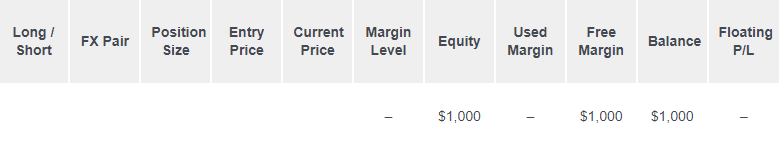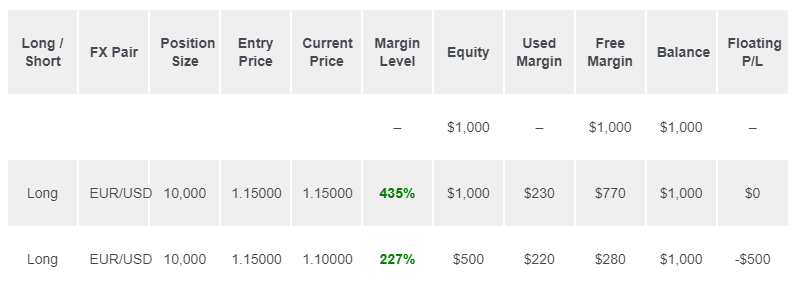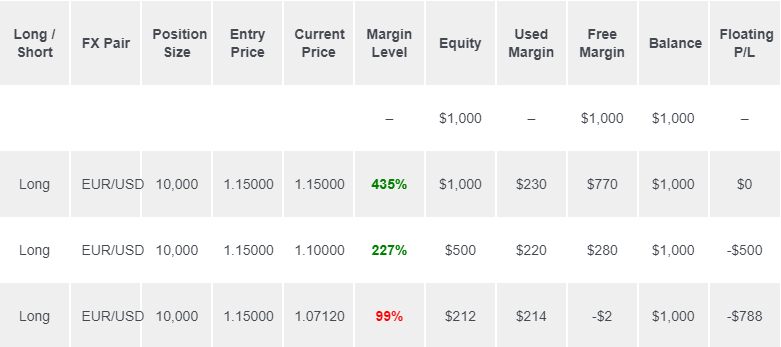TopAsiaFX

5 Oct, 2023

# Trading Scenario: Margin Call Level at 100% with No Separate Stop Out Level

In a trading scenario with a Margin Call Level at 100% and no separate Stop Out Level, when a trader's equity equals or falls below their used margin, the broker issues a margin call. Additional funds must be added to maintain open positions, preventing potential liquidation.Now, let's put your newfound knowledge of margin-related terms into practice by exploring trading scenarios involving different Margin Call and Stop Out Levels.

Various retail forex brokers and CFD providers have distinct margin call policies. Some exclusively employ Margin Calls, while others establish both Margin Call and Stop Out Levels.

In this example, we'll delve into a practical trading scenario with a broker that solely uses a Margin Call policy. This broker sets its Margin Call Level at 100% and does not have a separate Stop Out Level.

Let's see how your margin account fares when you find yourself in a trade that takes a turn for the worse.

Imagine you begin with an account balance of \$1,000.## Step 2: Determine the Required Margin

Suppose you decide to initiate a long position on EUR/USD at the rate of 1.15000, aiming to open a position with 1 mini lot (10,000 units). The Margin Requirement for this trade is 2%.

To determine the required margin for this position, take into account that the base currency is EUR. This mini lot corresponds to 10,000 euros, indicating that the position's Notional Value is €10,000.

Since your trading account is in USD, you'll need to convert the EUR value to USD to ascertain the trade's Notional Value.

• \$1.15 = €1

• \$11,500 = €10,000

The Notional Value amounts to \$11,500.

Now, let's calculate the Required Margin:

• Required Margin = Notional Value x Margin Requirement

• \$230 = \$11,500 x .02

Assuming your trading account is denominated in USD, given the 2% Margin Requirement, the Required Margin stands at \$230.

## Step 3: Calculate the Used Margin

With the exception of the recently initiated trade, there are no other open positions. Consequently, as there is only a SINGLE position open, the Used Margin is equal to the Required Margin.

## Step 4: Determine Equity

For the sake of this calculation, let's assume that the market has shifted slightly in your favor, and your position is currently at a breakeven point. This signifies that your Floating P/L is \$0.

To compute your Equity, use the following formula:

• Equity = Balance + Floating Profits (or Losses)

• \$1,000 = \$1,000 + \$0

Your account's Equity now totals \$1,000.

## Step 5: Calculate Free Margin

Now that you've established the Equity, you can proceed to calculate the Free Margin:

• Free Margin = Equity - Used Margin

• \$770 = \$1,000 - \$230

The Free Margin amounts to \$770

## Step 6: Determine Margin Level

With the Equity value determined, you can now calculate the Margin Level:

• Margin Level = (Equity / Used Margin) x 100%

• 435% = (\$1,000 / \$230) x 100%

The Margin Level stands at 435%.

At this juncture, these are the metrics that will be displayed in your trading platform for your account:## EUR/USD Drops 500 Pips!

Unexpectedly, reports of a zombie outbreak in Paris hit the headlines, causing EUR/USD to plummet by 500 pips, bringing it down to 1.10000.

Let’s assess the impact on your account.

### Used Margin

You'll notice that the Used Margin has changed.

Given the shift in the exchange rate, the Notional Value of the position has also altered. Consequently, the Required Margin needs to be recalculated.

Whenever the EUR/USD price experiences fluctuations, the Required Margin undergoes adjustments.

With EUR/USD now trading at 1.1000 (instead of 1.15000), let's determine the Required Margin needed to maintain the position open.

As our trading account is denominated in USD, we convert the EUR value to USD to establish the Notional Value of the trade.

• \$1.10 = €1

• \$11,000 = €10,000

The Notional Value amounts to \$11,000.

Previously, the Notional Value stood at \$11,500. The fall in EUR/USD signifies a weakening of the EUR, and since your account uses USD as its base currency, this reduction affects the position's Notional Value.

Now, let's calculate the Required Margin:

• Required Margin = Notional Value x Margin Requirement

• \$220 = \$11,000 x .02

Notably, the decrease in Notional Value has led to a reduction in the Required Margin.

Given that the Margin Requirement is 2%, the Required Margin now stands at \$220.

Previously, the Required Margin was \$230 (when EUR/USD was trading at 1.15000).

In this scenario, as you have only one open position, the Used Margin is equal to the new Required Margin.

### Floating P/L

EUR/USD has declined from 1.15000 to 1.10000, reflecting a 500-pip difference.

As you're trading 1 mini lot, a 1 pip movement equals \$1.

This translates to a Floating Loss of \$500.

• Floating P/L = (Current Price - Entry Price) x 10,000 x \$X/pip

• -\$500 = (1.1000 - 1.15000) x 10,000 x \$1/pip

### Equity

Your Equity now amounts to \$500.

• Equity = Balance + Floating P/L

• \$500 = \$1,000 + (-\$500)

### Free Margin

Your Free Margin is currently at \$280.

• Free Margin = Equity - Used Margin

• \$280 = \$500 - \$220

### Margin Level

Your Margin Level has decreased to 227%.

• Margin Level = (Equity / Used Margin) x 100%

• 227% = (\$500 / \$220) x 100%

Thankfully, your Margin Level remains above 100%, indicating that all is still well.

### Account Metrics## EUR/USD Drops Another 288 Pips!

EUR/USD takes another hit, plummeting by an additional 288 pips, bringing the rate down to 1.07120.

### Used Margin

Given the new EUR/USD rate of 1.07120 (as opposed to 1.10000), let's calculate the Required Margin necessary to maintain the position.

To do so, we convert the EUR value to USD to determine the Notional Value of the trade, as our trading account is in USD.

• \$1.07120 = €1

• \$10,712 = €10,000

The Notional Value is \$10,712.

Now, let's calculate the Required Margin:

• Required Margin = Notional Value x Margin Requirement

• \$214 = \$10,712 x .02

It's worth noting that the Required Margin has decreased in line with the reduced Notional Value.

Given the 2% Margin Requirement, the Required Margin now stands at \$214.

Previously, when EUR/USD traded at 1.10000, the Required Margin was \$220.

In this scenario, as you only have one open position, the Used Margin equals the new Required Margin.

### Floating P/L

EUR/USD has now dropped from 1.15000 to 1.07120, resulting in a significant 788-pip difference.

As you're trading 1 mini lot, a 1 pip movement corresponds to \$1.

This results in a Floating Loss of \$788.

• Floating P/L = (Current Price - Entry Price) x 10,000 x \$X/pip

• -\$788 = (1.07120 - 1.15000) x 10,000 x \$1/pip

### Equity

Your Equity has dwindled to \$212.

• Equity = Balance + Floating P/L

• \$212 = \$1,000 + (-\$788)

### Free Margin

Your Free Margin now stands at –\$2.

• Free Margin = Equity - Used Margin

• -\$2 = \$212 - \$214

### Margin Level

Your Margin Level has dipped to 99%.

• Margin Level = (Equity / Used Margin) x 100%

• 99% = (\$212 / \$214) x 100%

At this point, your Margin Level has fallen below the Margin Call Level!

### Account MetricsMARGIN CALL!

Upon the trade closure, two significant events unfold:

• Your Used Margin will be “released”.

• Your Floating Loss will be “realized”.

The Balance on your account will be adjusted to account for the Realized Loss.

With no remaining open positions, your Free Margin, Equity, and Balance will all align.

There will be no Margin Level or Floating P/L since all positions have been closed.

Now, let's examine how your trading account has transformed from start to finish.